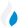•Trade Pi
•Trade
•Exchange
•Trade Pi
•Trade
•Exchange
\$1.1T
Total marketcap
\$75.64B
Total volume
39.69%
BTC dominance
•Trade Pi
•Trade
•Exchange

# matic to TWD Exchange Rate - 1 Polygon in TWD

38.580991797064
• BTC 0.000056
• ETH 0.0008
Vol [24h]
\$251,343.78

## matic to TWD converter

Exchange Pair Price 24h volume
BitoPro MATIC/TWD \$1.26 \$165.7K
Max Maicoin MATIC/TWD \$1.27 \$49K
Ace MATIC/TWD \$1.26 \$39.06K

## MATIC/TWD Exchange Rate Overview

Name Ticker Price % 24h 24h high 24h low 24h volume
Polygon matic \$1.25 -1.5712% \$1.34 \$1.24 \$1.05B

Selling 1 Polygon matic you get 38.580991797064 TWD.

Polygon Dec 27, 2021 had the highest price, at that time trading at its all-time high of \$2.92.

409 days have passed since then, and now the price is 43.29% of the maximum.

Based on the table data, the MATIC vs TWD exchange volume is \$251,343.78.

Using the calculator/converter on this page, you can make the necessary calculations with a pair of Polygon to TWD.

## Q&A

### What is the current MATIC to TWD exchange rate?

Right now, the MATIC/TWD exchange rate is 38.580991797064.

### What has been the Polygon to TWD trading volume in the last 24 hours?

Relying on the table data, the Polygon to TWD exchange volume is \$251,343.78.

### How can I calculate the amount of TWD? / How do I convert my Polygon to TWD?

You can calculate/convert TWD from MATIC to TWD converter. Also, you can select other currencies from the drop-down list.

## MATIC to TWD Сonversion Table

MATIC TWD
0.3 MATIC = 11.5 TWD
0.7 MATIC = 27.006 TWD
2 MATIC = 77.1 TWD
2 MATIC = 77.1 TWD
4 MATIC = 154.3 TWD
8 MATIC = 308.6 TWD
20 MATIC = 771.6 TWD
40 MATIC = 1,543.2 TWD
80 MATIC = 3,086.4 TWD
800 MATIC = 30,864.7 TWD
8000 MATIC = 308,647.9 TWD
80000 MATIC = 3,086,479.3 TWD
800000 MATIC = 30,864,793.4 TWD